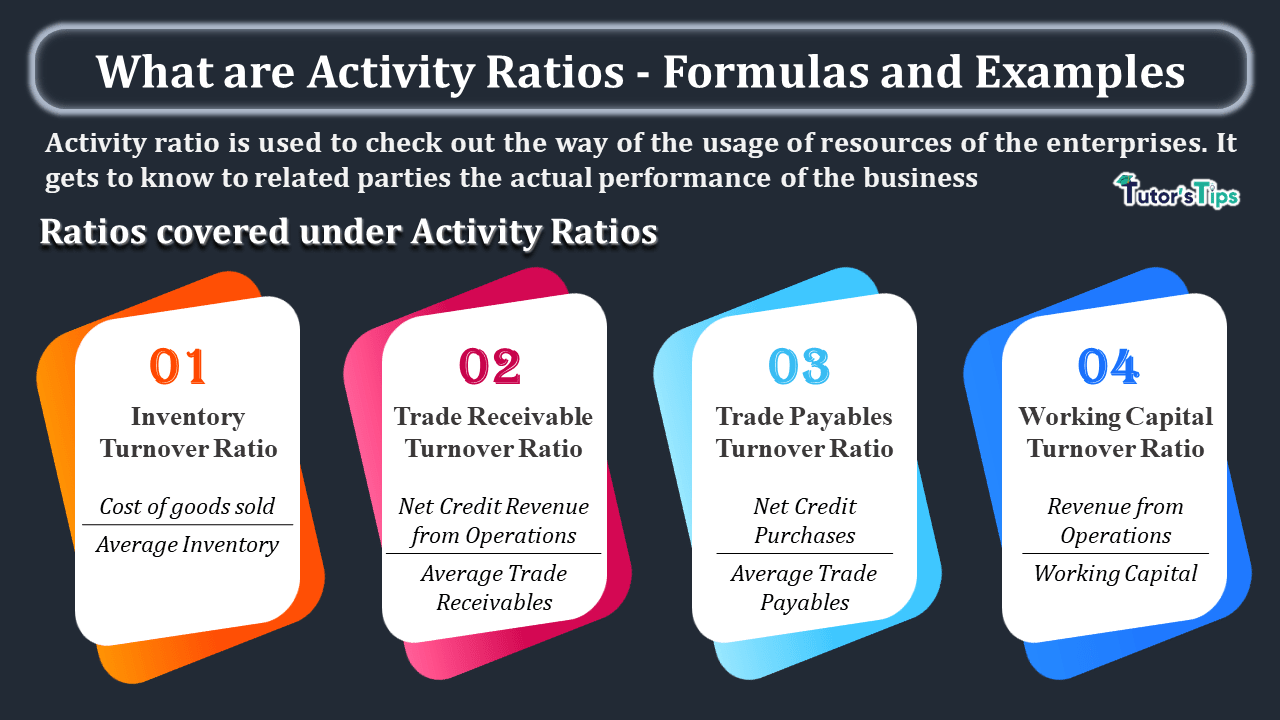# What are Activity Ratios – Formulas and ExamplesWhat are Activity Ratios - Formulas and Examples-min

Activity Ratios are used to know the performance of the resources of the business. It covers four types of ratios which are discussed in this article.

## Activity or Performance Ratios:

Activity ratio is used to check out the way of the usage of resources of the enterprises. It gets to know to related parties the actual performance of the business. These are also known as performance or turnover ratio.

## Why do we need to Calculate Activity Ratios?

Activity Ratios are needed to check out the actual usage of the resources of the business. These all ratios calculated in the form times. But these all are benefited differently some of them if low then these are better or some of them when these are high in time then these are better for the business. These are explained as under:

• Inventory Turnover Ratio is high then it is better for business because it shows the more sale from the less usage of inventory and vice versa.
• Trade Receivables Turnover Ratio is high then it is better for business because it shows the debts are collected promptly and vice versa.
• Trade Payables Turnover Ratio is Low then it is better for business because it shows the longer period to repay the payables and vice versa.
• Working Capital Turnover Ratio is high then it is better for business because it shows the efficient use of working capital and vice versa.

## The formula of Activity Ratios:

To Check out the liquidity of the business we can use the following three formulas:

### 1. Inventory Turnover Ratio:

The Inventory Turnover Ratio is used to know the relationship between the cost of goods sold and average inventory carries during the year of the business.

###### Formula to Calculate Inventory Turnover Ratio:
 Inventory Turnover Ratio = Cost of goods sold (cost of Revenue from Operation) Average Inventory
##### We will get ratio in times.

Now the question is how to calculate the cost of goods sold:

Total Revenue from an operation – Gross Profit

or

Total Revenue from an operation + Gross Loss

Or

Opening Inventory + Net Purchase + Direct Expenses – Closing Inventory

Now, the question is how to calculate the Average Inventory:

 Opening Inventory + Closing Inventory 2

### 2. Trade Receivable Turnover Ratio:

The Inventory Turnover Ratio is used to know the relationship between the Net Credit Sales and Average Trade Receivables.

###### Formula to Calculate Trade Receivable Turnover Ratio:
 Trade Receivable Turnover Ratio = Net Credit Revenue from Operations Average Trade Receivables
##### We will get ratio in times.

Now the question is how to calculate the Net Credit Revenue from Operations

Credit Revenue from Operations – Credit Sale Returns

Now, the question is how to calculate the Average Inventory:

Trade Receivables Includes: – Sundry Debtors and Bills Receivables

###### Formula to Calculate Debt Collection Period:
 = Numbers of Months(Days) in a year Trade Receivable Turnover Ratio

### 3. Trade Payables Turnover Ratio:

The Inventory Turnover Ratio is used to know the relationship between the Net Credit Purchases and Average Trade Payables.

##### We will get ratio in times.

Now the question is how to calculate the Net Credit Purchases

Credit Purchases – Credit Purchases Returns

Now, the question is how to calculate the Average Inventory:

##### Trade Receivables Includes: – Sundry Debtors and Bills Receivables
###### Formula to Calculate Average Payment Period:
 = Numbers of Months(Days) in a year Trade Payable Turnover Ratio

### 4. Working Capital Turnover Ratio:

The Working Capital Turnover Ratio is used to know the relationship between the working capital and total Revenue from an operation.

###### Formula to calculate Working Capital Turnover Ratio:
 Working Capital Turnover Ratio = Revenue from Operations Working Capital

OR

 Working Capital Turnover Ratio = Cost of Revenue from Operations Working Capital

We will get ratio in times.